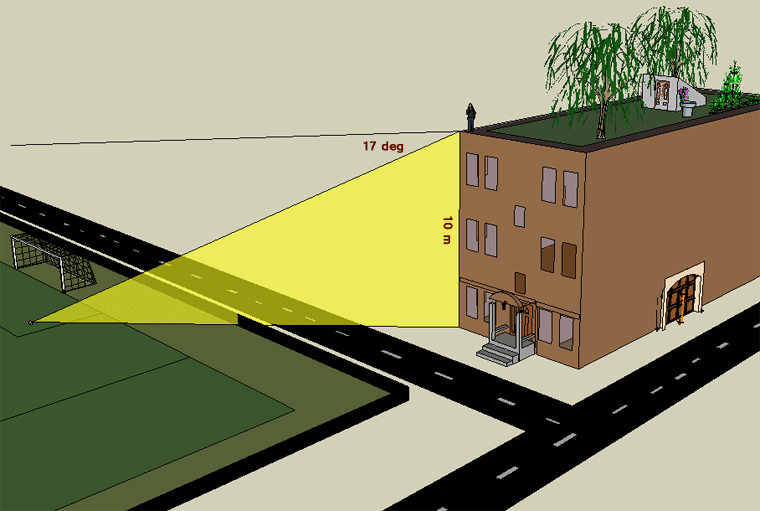SEARCH HOMEMath Central Quandaries & QueriesQuestion from Tosin, a student: the angle of depretion of a footboll from the top of a story building is 10m high is 17degree,find the distance of the boll frim the foot of the buildingHello Tosin. I hope this sketch I made is what you mean.The angle of depression (declination) is the angle made by the horizon (horizontal) and the line of sight somewhere below. The angle of inclination is the angle made by the horizon and the line of sight somewhere above. This means that the horizontal line through the air and the line along the ground between the building and the football are parallel.

One of the properties of parallel lines is that if a third line crosses them, then alternate interior angles are the same size. This means that the angle of depression from the rooftop to the football is the same as the angle of inclination from the football up to the rooftop. In other words, the angle of the yellow triangle at the football is also 17 degrees.

Assuming that the ground is flat and the building goes straight up, we have a right-angled triangle. So we can use a simple trigonometry ratio to find the length along the ground.

The TAN (tangent) function relates the opposite leg of a right triangle to the adjacent leg via the angle.

That is, if we let x = the unknown length along the bottom of the triangle, then

tan 17° = 10 / x.

Solve for x.

Hope this helps,
Stephen La Rocque.Math Central is supported by the University of Regina and The Pacific Institute for the Mathematical Sciences.